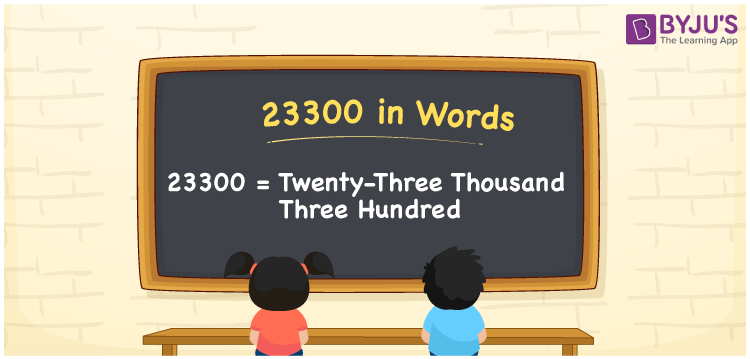# 23300 in Words

The number 23300 in words is written as Twenty-three thousand three hundred. Suppose a diary contains 23300 contact numbers; we can say, “The dairy has Twenty-three thousand three hundred contact numbers in it”. In this article, you will learn how to write the cardinal number 23300 in words using a place value chart, along with some facts about 23300.

 23300 in words Twenty-three thousand three hundred Twenty-three thousand three hundred in Numbers 23300

## 23300 in English words

Generally, we express numbers in words using the English alphabet. Thus, we can spell 23300 in English as “Twenty-three thousand three hundred”.## How to Write 23300 in Words?

To derive the word form of the number 23300, we need to create a five-column place value chart as the number 23300 has five digits. This can be done as follows.

 Ten thousand Thousands Hundreds Tens Ones 2 3 3 0 0

Here, ones = 0, tens = 0, hundred = 3, thousands = 3, ten thousands = 2

Let us expand these digits according to their place values.

2 × Ten thousand + 3 × Thousand + 3 × Hundred + 0 × Ten + 0 × One

= 2 × 10000 + 3 × 1000 + 3 × 100 + 0 × 10 + 0 × 1

= 20000 + 3000 + 300

= Twenty thousand + Three thousand + Three hundred

= Twenty-three thousand three hundred

Therefore, 23300 in words = Twenty-three thousand three hundred.

As we know, 23300 is a natural number that is the successor of 23299 and the predecessor of 23301.

23300 in words – Twenty-three thousand three hundred

Is 23300 an odd number? – No

Is 23300 an even number? – Yes

Is 23300 a perfect square number? – No

Is 23300 a perfect cube number? – No

Is 23300 a prime number? – No

Is 23300 a composite number? – Yes

## Frequently Asked Questions on 23300 in Words

Q1

### How do you write 23300 in words?

We can write the number 23300 in words as “Twenty-three thousand three hundred”.
Q2

### How to write Rs. 23300 in words on a cheque?

On a cheque, the amount Rs. 23300 can be written in words as Twenty-three thousand three hundred rupees only.
Q3

### Write the answer for 23300 – 15300 in words.

23300 – 15300 = 8000 Therefore, the value of 23300 – 15300, i.e. 8000 can be written in words as Eight thousand.